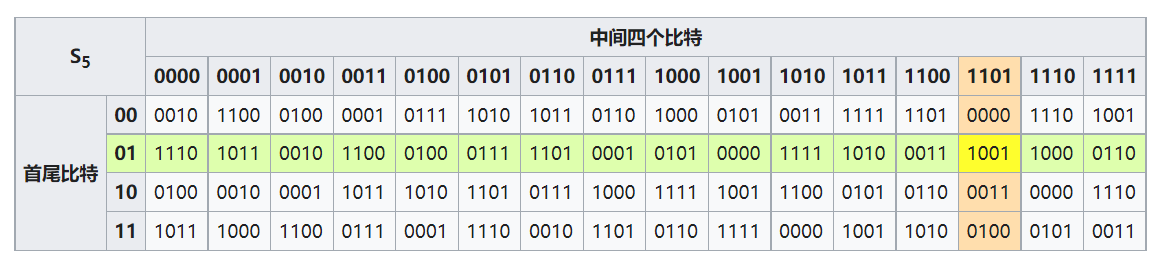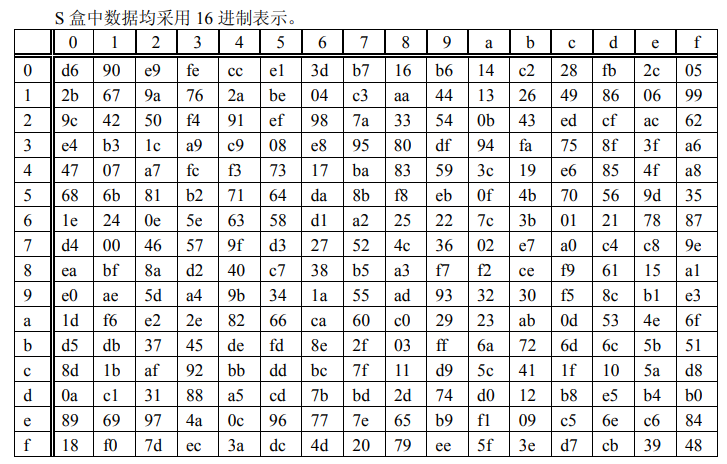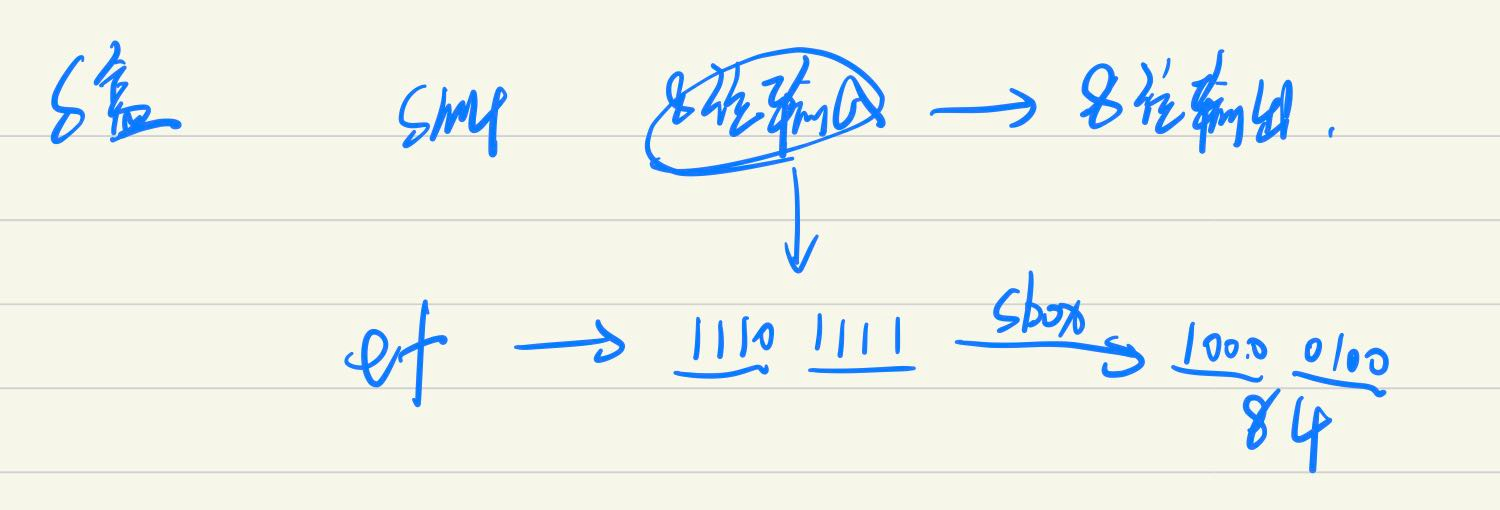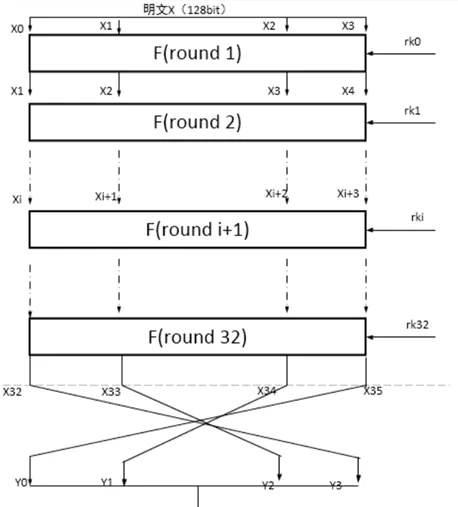SM4 算法

[TOC]

# 1. 国密 SM4 算法

SM4 分组密码算法是我国自主设计的分组对称密码算法，用于实现数据的加密/解密，以保证数据和信息的机密性。其算法公开，分组长度与密钥长度均为 128bit，加密算法密钥扩展算法都采用32 轮非线性迭代结构S 盒为固定的 8 比特输入 8 比特输出。

# 3. S 盒

## 3.1 DES S-box011011

100101

## 3.2 SM4 S-box

S 盒为固定的 8 比特输入 8 比特输出的置换# 4. 加/解密方式

SM4 算法加/解密算法的结构相同，只是使用轮密钥相反，其中解密轮密钥是加密轮密钥的逆序。

## 4.1 原理## 4.3 参数

• 输入 X

X0 X1 X2 X3 由 128 bit 拆分

• 加密密钥 MK, 128 bit

• 轮密钥 rk

共计 32 轮，32 个轮密钥

• 轮函数 F

每轮处理方式对应的函数

• 系统参数 FK, 4 个

• 固定参数 CK, 32 个

## 4.4 轮函数 F

$X_4 = F(X_0,X_1,X_2,X_3,rk) = X_0 \oplus T (X_1 \oplus X_2\oplus X_3\oplus rk_0)$

### 4.4.1 合成置换 T

$T(A_0,A_1,A_2,A_3) = L(\tau(A_0,A_1,A_2,A_3))$

#### 4.4.1.1 非线性变换

$B = \tau(A) = \tau(A_0,A_1,A_2,A_3) = (Sbox(A_0),Sbox(A_1),Sbox(A_2),Sbox(A_3))$

#### 4.4.1.2 线性变换 L

$C = L(B) = B \oplus (B <<< 2) \oplus (B <<< 10) \oplus (B <<< 18) \oplus (B <<< 24)$

## 4.5 加/解密算法

$X_{i+4} = F(X_i,X_{i+1},X_{i+2},X_{i+3},rk) = X_i \oplus T (X_{i+1} \oplus X_{i+2}\oplus X_{i+3}\oplus rk)$

$(Y_0,Y_1,Y_2,Y_3) = R(X_{32},X_{33}, X_{34}, X_{35}) = (X_{35},X_{34}, X_{33}, X_{32})$

## 4.6 密钥扩展算法

$(K_0,K_1,K_2,K_3) = (MK_0 \oplus FK_0,MK_1 \oplus FK_1,MK_2 \oplus FK_2,MK_3 \oplus FK_3)$

$rk_i 轮密钥，i = 0,1,2,...,31$

$rk_i = K_{i+4} = K_i \oplus T'(K_{i+1} \oplus K_{i+2} \oplus K_{i+3} \oplus CK_i)$

$T'(B_0,B_1,B_2,B_3) = L'(\tau(B_0,B_1,B_2,B_3))$

$L’(B) = B\oplus (B<<<13) \oplus (B <<< 23)$